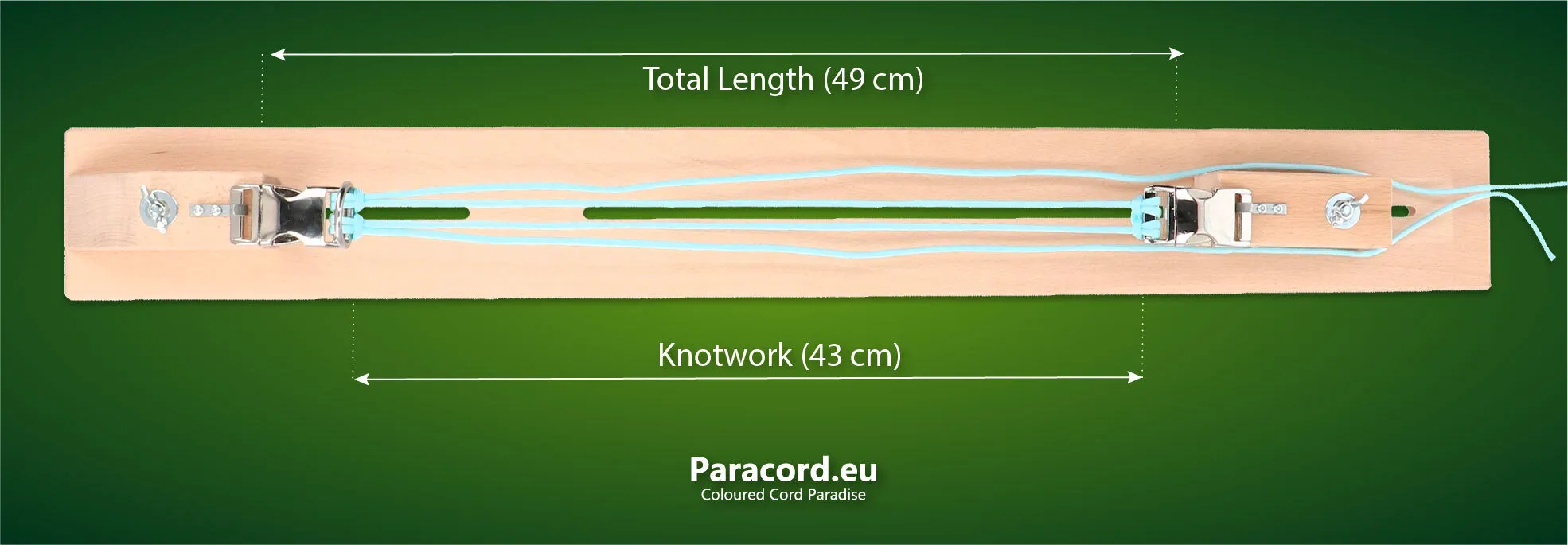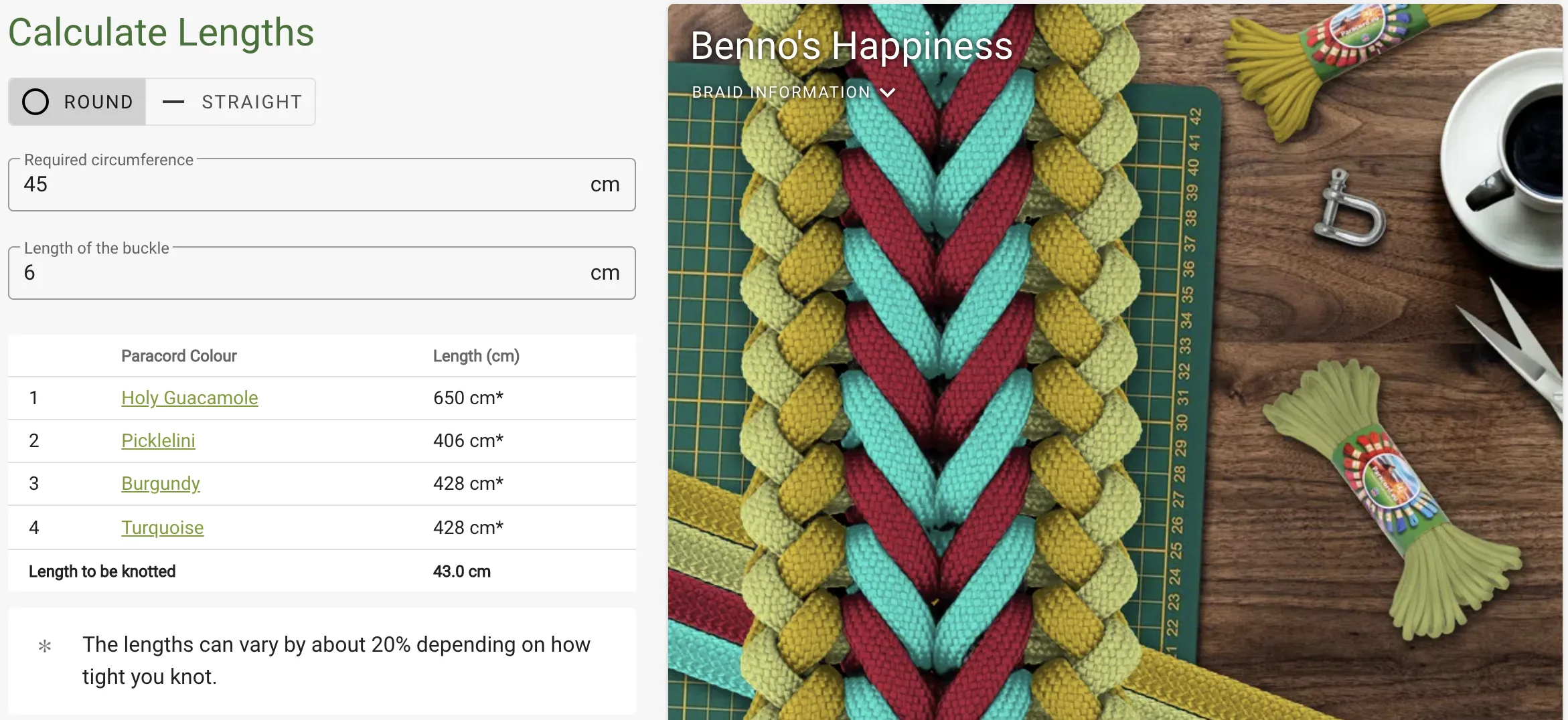By

# How do you use the jig formula?

The so-called jig formula is used by a large group of makers when making collars, armbands and other paracord projects which are based on a round shape. But how do you actually use this formula?

Determining the length of your knotwork depends on many different factors. You can find out more about these factors in the blog post about the thickness of a collar and in the post about measuring a dog's neck.

The jig formula can be a big help when determining the length for your collar. Use the formula to find out the total length of your project in centimetres.

## The jig formula

This is the formula:

Neck circumference + (3.14 * collar thickness) = total length

Let's take the Benno's Happiness as an example. This has a thickness of approx. 1.2 cm. The completed formula for a dog with a neck size of 45 cm looks like this:

45 + (3.14 * 1.2) = 48.77 = 49 cm

Imagine that you are using a 25 mm Alu-Max buckle for this collar. This is 6 cm long. You now deduct the length of the closure from the length for the complete collar.

49 cm - 6 cm = 43 cm

You now know that the total length of the project is 49 cm (including closure) and that the knotwork itself is 43 cm long.## How much cord do you need per colour?

Once you have determined the length to be knotted, you can calculate how much cord you need per colour. The required length of each cord depends on the knot you have in mind. The values given for this in manuals in turn always depend on the knotting style of the person who has calculated it. Therefore, it is always advisable to have slightly more than strictly necessary according to the instructions (e.g. 50 cm extra) when you are doing a knot for the first time.

### Calculate

How can you calculate how much you need yourself? You can do this by knotting a 10 cm piece and calculating how much cord you used for this. If you divide this by 10, then you know how much cord you need for 1 cm of knotwork. When you then start a new project, you can multiply that value by the intended length (length of the whole collar minus the length of the closure) to calculate how much you need.

Don't want to calculate this for yourself? Then you can use the calculator tool. This calculates the length you need to knot and how many cm you need per cord.